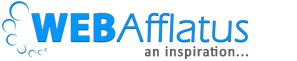# Finding Common Alphabet Between Two Names in C++/C

After getting more than 800 Hits to my previous post that is to find common alphabets between ‘AMITABH BACHCHAN’ and ‘RAJNIKANTH’ today I am going to post the code for finding common alphabets in C++. This work is done by my junior Ankit . He is in just 1st year. So I really appreciate his work and hope you will also like his codes. I am just posting on behalf of him. All credits goes go Ankit .

We have two names, say “AMITABH BACHCHAN ” and “RAJNIKANTH”. Our work is find common alphabet in these two names. The answer would be ‘AITHN’. i have written my code, if you find your code, more easier than mine, please do write in comment box. So lets go!

You can check the solution here. http://ideone.com/yAHm3Q

```#include<iostream>
using namespace std;
int main()
{
int len1, len2, x;
string s1="AMITABH BACHCHAN", s2="RAJNIKANTH", s="";
//if you want to take two names from the users then just define
//string s1,s1,s="";
//and remove the '//' from below lines.
//getline(cin,s1);
//getline(cin,s2);
len1= s1.length();
len2= s2.length();
for(int i=0;i<len1;i++)
{
for(int j=0;j<i;j++)
{
if(s1[i]==s1[j])
{
x=0;
break;
}
}
if(x==0)
{
x= 1;
continue;
}
for(int k=0;k<len2;k++)
{
if(s2[k]==s1[i])
{
cout<<s1[i]<<" ";
break;
}
}
}
return 0;
}
```

Thank You.

##### Signature

Deepesh SinghYou may also like.

1.harshit
2.gnanu